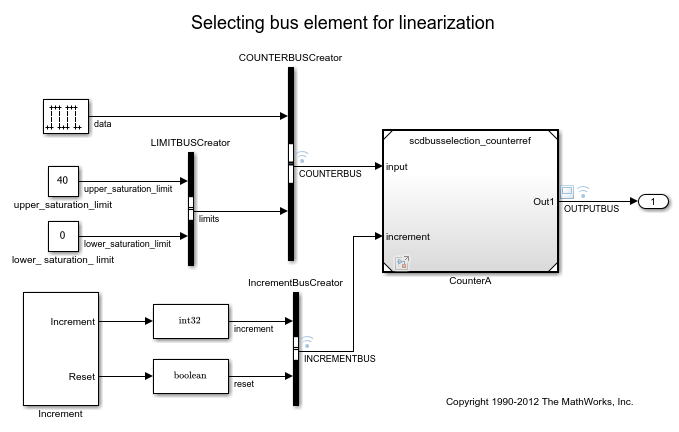Documentation

# linio

Create linear analysis point for Simulink model, Linear Analysis Plots block, or Model Verification block

## Syntax

``io = linio(block,port)``
``io = linio(block,port,type)``
``io = linio(block,port,type,[],busElement)``

## Description

example

````io = linio(block,port)` creates a linearization I/O object that represents an input perturbation analysis point for the signal that originates from the specified output `port` of a Simulink® `block`.```

example

````io = linio(block,port,type)` creates an analysis point of the specified `type`.```

example

````io = linio(block,port,type,[],busElement)` creates an analysis point for an element of a bus signal.```

## Examples

collapse all

```open_system('magball') ```To specify multiple analysis points for linearization, create a vector of linearization I/O objects.

Create an input perturbation analysis point at the output port of the Controller block.

```io(1) = linio('magball/Controller',1); ```

Create an open-loop output analysis point at the output of the Magnetic Ball Plant block. An open-loop output point is an output measurement followed by a loop opening.

```io(2) = linio('magball/Magnetic Ball Plant',1,'openoutput'); ```

View the specified analysis points.

```io ```
```1x2 vector of Linearization IOs: -------------------------- 1. Linearization input perturbation located at the following signal: - Block: magball/Controller - Port: 1 2. Linearization open-loop output located at the following signal: - Block: magball/Magnetic Ball Plant - Port: 1 ```

You can use these analysis points to linearize only the Magnetic Ball Plant subsystem. To do so, pass `io` to the `linearize` command or to an `slLinearizer` interface.

Unlike specifying analysis points directly in the Simulink model, when you create analysis points using `linio`, no annotations are added to the model.

```mdl = 'scdbusselection'; open_system(mdl) ```The `COUNTERBUS` signal, which originates from the COUNTERBUSCreator block, contains multiple bus elements.

Specify the `upper_saturation_limit` bus element as a linearization input. Select this element using dot notation, since it is within the nested `limits` bus.

```io = linio('scdbusselection/COUNTERBUSCreator',1,'input',[],... 'limits.upper_saturation_limit'); ```

## Input Arguments

collapse all

Simulink block from which the analysis point originates, specified as a character vector or string that contains its full block path. For example, to mark an analysis point at an output of the Controller block in the `magball` model, specify `block` as `'magball/Controller'`.

Output port of `block` from which the analysis point originates, specified as a positive integer.

`port` must be a valid port number for the specified `block`.

Analysis point type, specified as one of the following:

• `'input'` — Input perturbation

• `'output'` — Output measurement

• `'loopbreak'` — Loop break

• `'openinput'` — Open-loop input

• `'openoutput'` — Open-loop output

• `'looptransfer'` — Loop transfer

• `'sensitivity'` — Sensitivity

• `'compsensitivity'` — Complementary sensitivity

For more information on analysis point types, see Specify Portion of Model to Linearize.

Bus element name, specified as a character vector or string. When adding elements within a nested bus structure, use dot notation to access the elements of the nested bus. For an example, see Select Individual Bus Element as Analysis Point.

## Output Arguments

collapse all

Analysis point, returned as a linearization I/O object. Use `io` to specify a linearization input, output, or loop opening when using the `linearize` command. For more information, see Specify Portion of Model to Linearize.

Each linearization I/O object has the following properties:

PropertyDescription
`Active`

Flag indicating whether to use the analysis point for linearization, specified as one of the following:

• `'on'` — Use the analysis point for linearization. This value is the default option.

• `'off'` — Do not use the analysis point for linearization. Use this option if you have an existing set of analysis points and you want to linearize a model with a subset of these points.

`Block`

Full block path of the block with which the analysis point is associated, specified as a character vector.

`PortNumber`

Output port with which the analysis point is associated, specified as an integer.

`Type`

Analysis point type, specified as one of the following:

• `'input'` — Input perturbation

• `'output'` — Output measurement

• `'loopbreak'` — Loop break

• `'openinput'` — Open-loop input

• `'openoutput'` — Open-loop output

• `'looptransfer'` — Loop transfer

• `'sensitivity'` — Sensitivity

• `'compsensitivity'` — Complementary sensitivity

For more information on analysis point types, see Specify Portion of Model to Linearize.

`BusElement`

Bus element name with which the analysis point is associated, specified as a character vector or `''` if the analysis point is not a bus element.

`Description`

User-specified description of the analysis point, which you can set for convenience, specified as a character vector.

## Alternative Functionality

### Linear Analysis Tool

You can interactively configure analysis points using the Linear Analysis Tool. For more information see, Specify Portion of Model to Linearize in Linear Analysis Tool.

You can also specify analysis points directly in a Simulink model. When you do so, the analysis points are saved within the model. For more information, see Specify Portion of Model to Linearize in Simulink Model.

### `slLinearizer` and `slTuner` Interfaces

If you want to obtain multiple open-loop or closed-loop transfer functions from the linearized system without recompiling the model, you can specify linear analysis points using an `slLinearizer` interface. For more information, see Mark Signals of Interest for Batch Linearization. Similarly, if you want to tune a control system and obtain multiple open-loop or closed-loop transfer functions from the resulting system, you can specify linear analysis points using an `slTuner` interface. For more information, see Mark Signals of Interest for Control System Analysis and Design.